# Evaluation

## Comparison of CFD calculations with Experiments

6.1 Test Case 1 - σ = 0.286

The ESDU CFD results generated using CFX 5 are compared with the experimental and numerical data produced by Durst and co-workers in Figs 3-10. The ESDU CFD results predict the main flow features observed and measured experimentally, qualitatively and quantitatively. At a distance from the contraction plane equal to 1-2 × D the axial velocity profiles start to deform with higher values in the central volume of the pipe. For ReD ≥ 125 the velocity profile downstream of the contraction displays a typical overshoot with a peak close to the wall and a flat region in the central section. This agrees with Durst and co-workers work, but does not agree with Kelsey (1971) who reported the velocity overshoot to occur for ReD ≥ 50 in his numerical work for contractions with 1 ≤ β ≤ 8, and 0.01 ≤ ReD ≤ 500. The ESDU CFD predictions of the upstream separation decreases in size for ReD < 100 and then it increases. The downstream separation appears for ReD > 300 and its size increases with ReD. These flow features are also well predicted by the numerical work by Durst and co-workers.

Comparisons of the ESDU CFD predictions with LDA velocity measurements (see Figs 3-4) show that, for ReD<300 (no downstream separation), CFX predicts well the axial velocity components. However, the radial velocity components (Fig.5) are under-predicted by up to 40%. CFX radial velocity results are very close to the Durst and co-workers numerical results. For ReD>300, CFX results under-predict by about 5% velocity peaks at high Re in the velocity overshoot region (see Fig. 6). Durst and co-workers found similar discrepancies, although their numerical results improved with the mesh refinement used in Buckle and Durst (1993). The largest discrepancy is found at ReD=1213 (Fig. 6), and can be partly due to the flow regime being in the transition region.

Comparisons of pressure profiles in Fig. 7 show that ESDU CFD results are close to Durst and co-workers results, being slightly over-predicting. Comparisons of the upstream separation size in Fig. 8 show that ESDU CFD predictions display similar trends to Durst and co-workers numerical results. However, CFX predictions of the upstream separation length are lower at higher ReD, while the separation height is higher. This could be due to the relatively coarser mesh and shorter large- and small-diameter tubes (L=D and l=1.3×D) used by Durst and co-workers. CFD calculations carried out by the authors for similar mesh size and the same short tube lengths have shown at ReD=1213 differences with Durst and Loy numerical results for the upstream and downstream separation sizes up to 5% and 10%, respectively. Comparisons of the downstream separation size in Fig. 9 show that ESDU CFD predictions are within the experimental error ± 30%, and are closer to the experiments than Durst and co-workers numerical results.

Comparisons of ESDU CFD predictions with the ESDU correlation for the pressure loss coefficient KL are shown in Fig. 10. The CFD predictions of KL in laminar flow are in general within the reported correlation error (Kaye and Rosen, 1971). For ReD<200, the CFD predictions are up to 70% lower than the ESDU correlation. This could be due to a high experimental error of pressure drop measurements at low Re. Although Kaye and Rosen (1971) reported an error for their correlation of ± 20 % over the laminar flow Re range, the minimum precision of their pressure measurements is 0.5 Pa, which could imply a significant overestimation of the pressure measurements at low Re. CFD calculations have been performed by the authors for the same test geometry used by Kaye and Rosen with lowest and highest contraction ratios (σ=0.629 and 0.026). The predicted inlet pressure values at ReD=23 were about 1 Pa for σ=0.026, and 0.2 Pa for σ=0.629 with an error of 30% and -83% from their correlation, respectively. This seems to indicate that the ESDU CFD predictions error depends on the inlet pressure condition and is lower at higher pressure. Also, it could imply that the Kaye and Rosens correlation error at ReD=23 can be estimated as 50% minimum (i.e. for a inlet pressure of 1 Pa the minimum, 0.5 Pa maximum measurement error). Comparisons of a numerically predicted pressure loss coefficient are reported by Durst and co-workers for β=8 with the experimental data published by Sylvester for and Rosen (1970). These show that CFD under-predicts K</nowiki>L consistently by an estimated 40% to 50%. This is thought (Durst and Loy, 1985) to be due to grid refinement (grid size of 4740).

In turbulent flow regime, the ESDU CFD predictions are lower than the ESDU correlation data for all turbulent models under investigation. Bullen experimental predictions of KL for a similar contraction ratio (σ=0.297) are upto 15% lower than the ESDU correlation but within its estimated error. Predictions using the k-ε model with Scaleable Wall Function are within the estimated error and are closest to the ESDU correlation (within 20%); predictions using the k-ω model with Automatic near-wall treatment are nearly within the estimated error (-22% maximum difference) and are closest to Bullens measurements for KL on average (within 10%); predictions using the SST model with Automatic near-wall treatment are about 25% below the ESDU correlation (-5% the estimated error). Predictions in the transition region using SST and k-ω models with Automatic near-wall treatment are reasonably filling the gap between laminar and turbulent flow regions.

The ESDU CFD predictions of the upstream and downstream separation regions for 23 ≤ ReD ≤ 106 are shown in Fig. 11 and 12. Predictions using SST and k-ω models with Automatic near-wall treatment display similar trends and get very close for ReD> 105. These trends show an increase of the separation length and height up to ReD=104. For ReD> 104, the upstream separation size decreases. This can be due to the appearance of a small vortex at the corner of the contraction plane at this flow condition, which can also affect the size of the downstream separation. In fact, the downstream separation length increases up to ReD=2000, it then decreases up to ReD=104-105, and it increases again at higher ReD. Predicted values of this length using the SST model are about 10% lower than those using the k-ω model. The downstream separation height increases monotonically with Re and tends to similar values for the SST and k-ω models. Predictions using the k-ε model with Scaleable Wall Function are not reliable as the upstream separation regions are much smaller than expected (separation sizes predictions do not connect up with laminar flow predictions). At ReD=104 no upstream separation is predicted; at higher ReD, predictions seem to improve with increase in ReD with differences from the SST and k-ω models predictions at ReD=106 of 60% and 30% for Ls1x and Ls1r, respectively. Downstream separation region are also smaller than expected, although a small separation is predicted for ReD=104. Predictions for this separation size also improve with ReD, and differences from the SST and k-ω models predictions at ReD=106 are 3% and 10% for Ls2x and Ls2r, respectively. From these results it can be concluded that the SST and k-ω models with Automatic near-wall treatment predict well flow separation details but under-predict KL.

Figure 3. Comparison of axial velocities predicted by ESDU using CFX 5 with Durst and Loy (1985) LDA measurements at various locations and ReD=23, (a) upstream, and (b) downstream of the contraction.

Figure 4. Comparison of axial velocities predicted by ESDU using CFX 5 with Durst and Loy (1985) LDA measurements at various locations and ReD=196, (a) upstream, and (b) downstream of the contraction.

Figure 5. Comparison of radial velocities predicted by ESDU using CFX 5 with Durst and Loy (1985) LDA measurements and numerical results at various locations and ReD=196, (a) upstream, and (b) downstream of the contraction.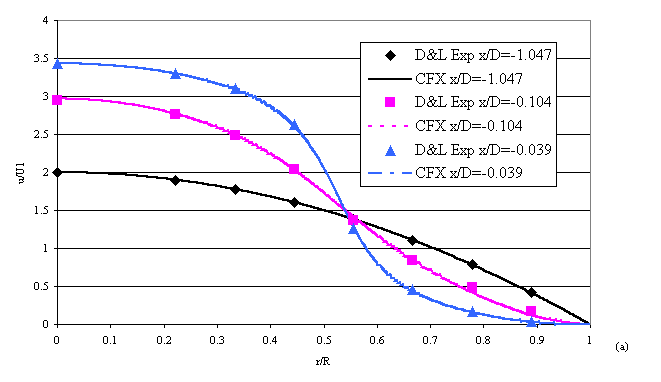Figure 6. Comparison of axial velocities predicted by ESDU using CFX 5 with Durst and Loy (1985) LDA measurements and numerical results at various locations and ReD=1213, (a) upstream, and (b) downstream of the contraction.

Figure 7. Comparison of pressure profiles predicted by ESDU using CFX 5 with Durst and Loy (1985) numerical results at various locations and ReD=563, (a) upstream, and (b) downstream of the contraction.

Figure 8. Comparison of upstream separation length Ls1x and height Ls1r predicted by ESDU using CFX 5 with Durst and Loy (1985) numerical results at different ReD.

Figure 9. Comparison of downstream separation length Ls2x and height Ls2r predicted by ESDU using CFX 5 with Durst and Loy (1985) measurements and numerical results at different ReD.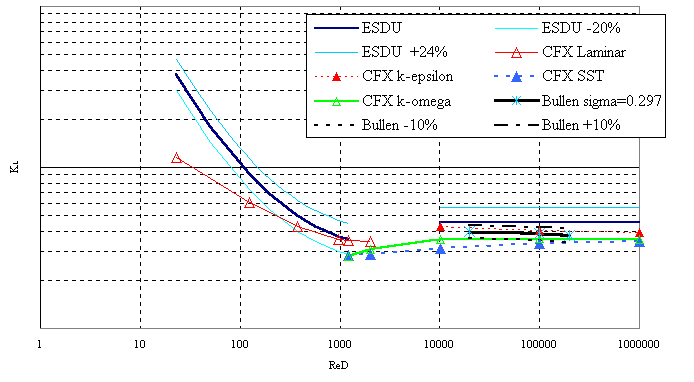Figure 10. Comparison of pressure loss coefficient KL predicted by ESDU using CFX 5 with ESDU correlation and Bullen et al. (1996) experimental predictions at different ReD.

Figure 11. ESDU CFD predictions of upstream separation length Ls1x (a), and height Ls1r (b) at different ReD.

Figure 12. ESDU CFD predictions of downstream separation length Ls2x (a), and height Ls2r (b) at different ReD
6.2 Test Case 2 - '
σ' = 0.332

The ESDU CFD predictions for this Test Case are compared with Bullen and co-workers experimental and CFD results for ReD =1.54×105 in Figs 13-16. The flow features and their development with increasing Re are similar to those for Test Case 1.

Comparisons of axial velocity with LDA measurements show that the ESDU CFD predictions upstream of the contraction are very close to the experiments (Fig. 13(a)). Results using the SST and k-ω models with Automatic near-wall treatment are very close over the radius, and are the closest to the measurements. Results using the k-ε model with Scaleable Wall Function differ slightly at the pipe wall at x/D = -0.1. The numerical results by Bullen and co-workers using the k-ε model with Standard Wall Function predict the velocity profile closest to the contraction only qualitatively. Bullens measured a negative velocity at x/D=-0.1 and y/R=0.94. The ESDU CFD predictions at this location produced: the SST model a negative velocity at y/R=0.94, the k-ω model a negative velocity y/R=0.97. The k-ε model did not produce a negative velocity at this location. Comparisons downstream of the contraction plane (Fig.13(b)) show that the ESDU CFD results predict well (within about 5%) the velocity overshoot in the vicinity of the contraction plane (x/D=0.15). Some discrepancies close to the wall can be seen which could be due to the experiments not being capable of measuring flow separation details in this region. In fact, comparisons of the core flow extent in the downstream separation region in Fig. 16 show that both the SST and the k-ω models predict well the separation region. ESDU CFD results using the k-ε model under-predict the velocity overshoot and the separation region extent. However, they are much closer to the experiments than Bullen CFD results; these predict a velocity overshoot, but do not predict flow separation (i.e. u>0 at all r/R) at x/D=0.15. Bullens measured a velocity overshoot at x/D=0.125 and y/R=0.8 of 1.18 in magnitude. The ESDU CFD predictions at this location produced: the SST model an overshoot of 1.16 at y/R=0.79, the k-ω model an overshoot of 1.14 at y/R=0.76, and the k-ε model an overshoot of 1.11 at y/R=0.69. ESDU CFD predictions at x/D=1 near the pipe wall differ from the experiments somewhat. This can be due to slow flow recovery against an adverse pressure gradient, which is renowned in CFD predictions.

Comparisons of the radial velocity LDA measurements with the ESDU CFD predictions (Fig. 14) show that upstream of the contraction plane (x/D=-0.1) all turbulent models generate similar results. These over-predict velocity magnitude (by about 15%) but produce the correct trend. Bullen numerical results predict the correct trend with a higher over-prediction of the velocity magnitude. Comparisons downstream of the contraction plane (x/D=0.125) show that ESDU CFD results using the SST and the k-ω models with Automatic near-wall treatment are very close to the experiments; results using the k-ε model with Scaleable Wall Function predict lower velocity magnitudes and positive values close to the wall. These are close to Bullen numerical results obtained using the standard k-ε model.

Comparisons of the centre line velocity with ESDU CFD results (Fig. 15) show that trends are predicted well, but the peak magnitude downstream of the separation is under-predicted for all turbulent models. Results using the SST and the k-ω models are very similar up to x/D = 5. For x/D > 5 results using the k-ω and the k-ε models show a faster recovery (at about x/D=10) than the SST model (at about x/D=20). The experimental data seem to indicate a recovery between x/D=10 and 15. Comparisons of the core flow extent in the downstream separation region estimated by Bullen and co-workers with ESDU CFD predictions are in Fig.16. Results using the SST model are the closest to the experiments within a reattachment point within +10%, followed by the k-ω with a reattachment point within about 10%. Results using the k-ε model under-predict the core extent by about 30%, both in the axial and radial directions.

Comparisons of the pressure loss coefficient KL with the ESDU correlation and the experimentally predictions of Bullen and co-workers are in Fig. 17. In laminar flow regime, as in Test Case 1 the ESDU CFD results under-predict the ESDU correlation up to 70% for ReD<400. Predictions for ReD>400 are within the reported correlation error (Kaye and Rosen, 1971). As discussed in Section 6.1, at low Re the pressure measurements carried out by Kaye and Rosen to derive KL could have a significant experimental error, which, although difficult to quantify, could imply an error in the KL correlation greater than 50%. In turbulent flow regime, results using all turbulent models under-predict KL (both ESDU and Bullens predictions). Results using the k-ε model with Scaleable Wall Function are closest to the ESDU correlation and Bullens predictions, followed by the k-ω model with Automatic near-wall treatment, and are within the estimated error for the ESDU correlation (-10% on average) and Bullens predictions (-10% on average). Results using the SST model with Automatic near-wall treatment are on average about 23% below the ESDU correlation, and 12% below Bullens prediction. Results using the k-ω model with Automatic near-wall treatment are about 22% below the ESDU correlation and -8% below the Bullens predictions.

The ESDU CFD predictions of the upstream and downstream separation sizes are plotted in Figs 18 and 19. The trends are similar to those for Test Case 1. In laminar flow, the upstream separation size (Fig.18) decreases with ReD for ReD<100, and increases linearly for ReD>100. In turbulent flow, results for the SST and k-ω models with Automatic near-wall treatment display similar trends and tend to converge at high ReD. Both models predict an increase of the separation size up to ReD=104, and a decrease at higher ReD. Results using k-ε model with Scaleable Wall Function are not reliable as the upstream and downstream separation sizes are much lower than expected. Differences between the k-ε model with Scaleable Wall Function and the SST and k-ω models with Automatic near-wall treatment are similar to those discussed in Section 6.1 for Test Case 1. In laminar flow, the size of the downstream separation increases with Re (Fig. 19). In turbulent flow, both the SST and k-ω models show an increase of the separation length up to about ReD=104. For ReD>104, it decreases slightly for the SST model, while increases for the k-ω model. Values at high Re tend to converge. The separation height increases with Re in laminar and turbulent flow.

In conclusion, the results from the above Test Cases have shown that, in turbulent flow regime, the SST model with Automatic near-wall treatment is able to capture the flow details in pipes with sudden contraction very well, qualitatively and quantitatively. However, predictions of the pressure loss coefficient are slightly below the experimental error. The k-ω model with Automatic near-wall treatment, can predict the flow details well and can predict pressure loss coefficient within the experimental error. The k-ε model with Scaleable Wall Function can predict pressure loss coefficient within the experimental error, but cannot predict the flow details accurately. However, the poor performance of the k-ε model for predicting the flow separation sizes (do not connect to laminar flow predictions) is not attributable to the model itself but to the Wall Function used even though it is a Scaleable one. In transitional flow regime, both the SST and k-ω models with Automatic near-wall treatment reasonably predict the flow details and separation sizes. Their prediction of the pressure loss coefficient fits well between the laminar and turbulent flow predictions. However, the k-ω model with Automatic near-wall treatment is probably most suitable, as its predictions in turbulent flow are closest to the experiments and those in transitional flow are closest to the high-Re laminar flow predictions. These findings are partly in agreement with a recent work by Zhang and Kleinstreuer (2003) who compared experimental measurements of low Re turbulent flows in constricted conduits in all flow regimes with four low-Re (LRN), i.e. with near wall treatment, turbulent models: the LRN k-ε (Jones and Launder, 1973), the RNG k-ε, the Menter k-ω (Menter, 1994), and the LRN k-ω (Wilcox, 1998). They established that: the LRN k-ω is the most suitable for simulating laminar-transitional-turbulent flows in constricted tubes, in agreement with Wilcox (1998) who showed that the LRN k-ω can predict transitional flow.

Figure 13. Comparison of axial velocities predicted by ESDU using CFX 5 with Bullen et al. (1990) LDA measurements and FLUENT results at various locations and ReD=1.54×105, (a) upstream, and (b) downstream of the contraction.

Figure 14. Comparison of radial velocities predicted by ESDU using CFX 5 with Bullen et al. (1990) LDA measurements and FLUENT results at two locations and ReD=1.54×105.

Figure 15. Comparison of centre line velocities predicted by ESDU using CFX 5 with Bullen et al. (1996) LDA measurements for ReD=1.54×105.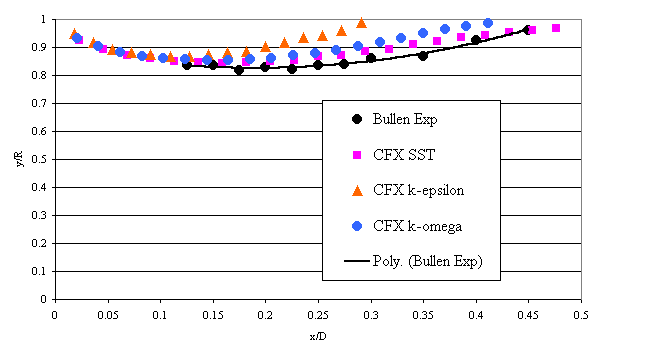Figure 16. Comparison of core flow extent in downstream separation predicted by ESDU using CFX 5 with Bullen et al. (1990) measurements at ReD =1.54×105.

Figure 17. Comparison of pressure loss coefficient predicted by ESDU using CFX 5 with ESDU correlation and Bullen et al. (1996) experimental predictions at different ReD.

Figure 18. ESDU CFD predictions of upstream separation length Ls1x (a), and height Ls1r (b) at different ReD.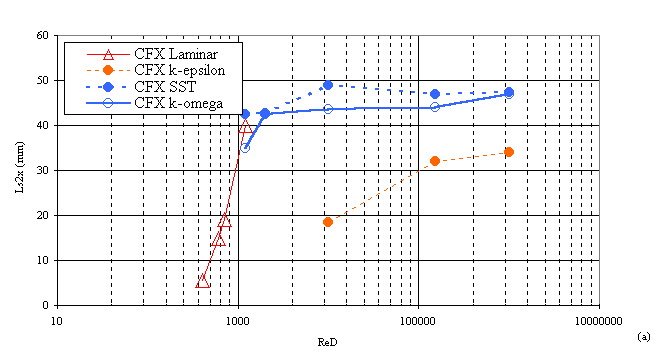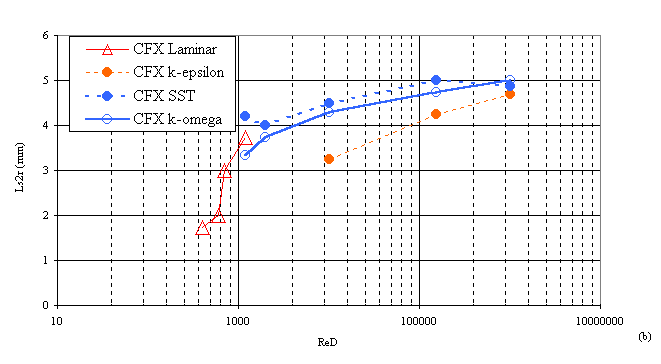Figure 19. ESDU CFD predictions of downstream separation length Ls2x (a), and height Ls2r (b) at different ReD.